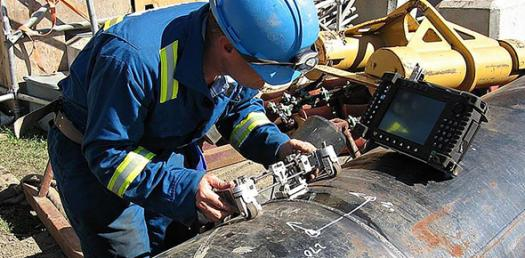# Corrosion Engineering Quiz: How Well Do You Know Corrosion Engineering?

20 Questions | Total Attempts: 474SettingsA list of questions related to corrosion engineering which are surely going to boggle your heads !

• 1.
Rusting of iron is a typical example for
• A.

Chemical corrosion

• B.

Electrochemical corrosion

• C.

Physical reaction

• D.

None of these

• 2.
Pitting factor of unity represents ____ corrosion.
• A.

Uniform

• B.

Galvanic

• C.

Inter granular

• D.

Fretting

• 3.
Passivity in stainless steel is due to the formation of
• A.

Sulphide film

• B.

Carbide film

• C.

Oxide film

• D.

Chloride film

• 4.
Iron pipe carrying water is more anodic to copper. This is due to
• A.

Concentration cell

• B.

Galvanic cell

• C.

Electrolytic cell

• D.

Leclanche cell

• 5.
The tip and morphology of the crack due to SCC phenomenon will be
• A.

Sharp and transgranular

• B.

Blunt and intergranular

• C.

Blunt and transgranular

• D.

None of these

• 6.
Which of the following metal can be used for cathodic protection of steel
• A.

Al

• B.

Cu

• C.

Ag

• D.

Au

• 7.
In zinc – aluminium galvanic couple, when exposed to acidic solutions
• A.

Zinc dissolves

• B.

Aluminium dissolves

• C.

Both dissolve

• D.

None of these

• 8.
The equation for uniform corrosion is given by :
• A.

Mpy = 534/(DAT)

• B.

Mpy = 584/(DAT)

• C.

Mpy = (DAT) / 534

• D.

Mpy = (DAT) / 584

• 9.
Typical example for anodic metallic coating is
• A.

Zn on Steel

• B.

Cu on Steel

• C.

Au on steel

• D.

All of these

• 10.
Pourbaix diagram is a graphical plot of
• A.

E Vs pH

• B.

E Vs Log i

• C.

E Vs time

• D.

Current Vs Potential

• 11.
The terminology used for uniform corrosion of nickel and nickel base alloys is
• A.

Tarnishing

• B.

Fogging

• C.

Dulling

• D.

None of these

• 12.
Knife line attack in IGC refers to
• A.

Low temperature HAZ

• B.

High temperature HAZ

• C.

Weld zone

• D.

Base metal

• 13.
Pilling – Bedworth ratio is pertinent to
• A.

Internal oxidation

• B.

High temperature sulphidation

• C.

Catastrophic oxidation

• D.

High temperature oxidation

• 14.
When a non-metallic material forms a crevice on metallic surface it is known as
• A.

Pitting

• B.

• C.

Uniform

• D.

Fretting

• 15.
The sensitization caused in stainless steel for IGC to occur is _____ to corrosive medium
• A.

The prerequisite before exposure

• B.

Post treatment after exposure

• C.

Mere thermal cycle without exposure

• D.

None of these

• 16.
The main cause for the IGC attack in SS is the formation of ____ at the grain boundary.
• A.

Chromium carbide precipitate

• B.

Titanium carbide

• C.

Molybdenum carbide

• D.

Tungsten carbide

• 17.
Experimental setup for stress corrosion cracking should involve ___ application of ultimate tensile stress.
• A.

100%

• B.

0%

• C.

Less than 100%

• D.

None of these

• 18.
The formation of horse-shoe pit is one of the methods of recognizing the occurrence of ____ corrosion.
• A.

Pitting

• B.

Galvanic

• C.

Erosion

• D.

Intergranular

• 19.
The phenomenon of corrosion that occurs on rail roads is _____
• A.

Crevice

• B.

Corrosion fatigue

• C.

High temperature oxidation

• D.

Fretting

• 20.
Catastrophic oxidation is exhibited by metals obeying ____ rate law kinetics.
• A.

Logarithmic

• B.

Parabolic

• C.

Linear

• D.

Cubic

Related TopicsBack to top